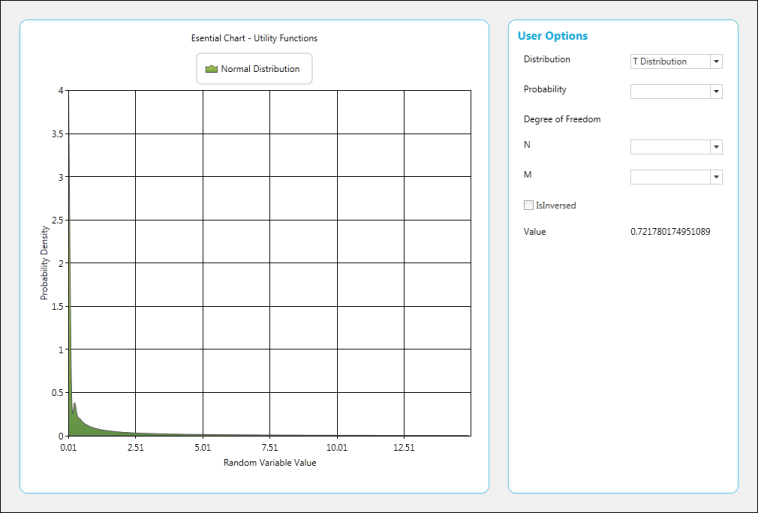# Statistical Formula and Utility Functions in WPF Chart (Classic)

5 May 202114 minutes to read

This feature allows the user to calculate basic statistical functions that include mean, median, standard deviation, variance, variance based estimator, variance unbased estimator, correlation coefficient and covariance, ANOVA, T-test, Z-test, and F-test performed based on sample series, and utility functions like normal distribution, T-cumulative distribution and F-cumulative distribution.

## Use Case Scenarios

1. This feature supports built-in statistical formulas.
2. The curve in the graph can be drawn based on normal distribution, T-cumulative distribution, and F-cumulative distribution.
3. Basic functions like mean, median, and standard deviation can be obtained from the series drawn.

## Methods

Method Description Parameters Return Type
Mean Returns the mean value of the x values of the series. Series Double
Mean Returns the mean of y values. Series, Yindex(int) Double
VarianceUnbiasedEstimator Estimates the variance for the x values of the series. Series Double
VarianceUnbiasedEstimator Estimates the variance for the y values of the series. Series,Yindex(int) Double
VarianceBiasedEstimator Estimates the variance of the sample. Series Double
VarianceBiasedEstimator Estimates the variance for the y value of the series. Series, Yindex(int) Double
Variance The following code samples demonstrate how to get the variance of the data points in a series. Series,sampleVariance(bool) Double
Variance The following code samples demonstrate how to get the variance of the y-value data points in a series. Series,Yindex(int),sampleVariance(bool) Double
StandardDeviation This method determines the standard Deviation for x values of the series. Series,sampleVariance(bool) Double
StandardDeviation This method determines the standard deviation for y values of the series. SeriesYindex(double)sampleVariance(bool) Double
Covariance Returns the average of the product of deviations of the data points from their respective means. Series1,Series 2 Double
Covariance Returns the average of the product of deviations of the data points from their respective means based on y values. Series1,Series 2,Yindex(int) Double
Correlation Measures the relationship between two data sets that are scaled to be independent of the unit of measurement. This correlation method returns the covariance of two data sets divided by the product of their standard deviations, and always ranges from -1 to 1. Series1,Series2 Double
Correlation Measures the relationship between two data sets that are scaled to be independent of the unit of measurement. This correlation method returns the covariance of two data sets divided by the product of their standard deviations, and always ranges from -1 to 1. Series1,Series2, yIndex(double) Double
Median Calculates the median of the points stored in a series. Series Double
Median Calculates the median of the points stored in a series. Series,yIndex(int) Double
ZTest This method performs a Z-test for two groups of data and returns the results using a ZTestResult object. (double) hypothesizedMeanDifference, (double) varianceFirstGroup, (double) varianceSecondGroup, (double) probability, (ChartSeries) firstInputSeries, (ChartSeries) secondInputSeries ZTestResult
ZTest This method performs a Z-test for two groups of data and returns the results using a ZTestResult object, for Y values. (double )hypothesizedMeanDifference, (double) varianceFirstGroup, (double) varianceSecondGroup, (double) probability, (ChartSeries )firstInputSeries, (ChartSeries )secondInputSeries,(Int) yIndex ZTestResult
TTestEqualVariances This method performs a T-test for two groups of data and assumes equal variances between the two groups (i.e. series) for the x values. (Double) hypothesizedMeanDifference, (double) probability, (ChartSeries)firstInputSeries, (ChartSeries) secondInputSeries TTestResult
TTestEqualVariances This method performs a T-test for two groups of data and assumes equal variances between the two groups (i.e. series) for the y values. (Double) hypothesizedMeanDifference, (double) probability, (ChartSeries)firstInputSeries, (ChartSeries) secondInputSeries,(int)yIndex TTestResult
TTestUnEqualVariances This method performs a T-test for two groups of data and assumes unequal variances between the two groups (i.e. series). (Double) hypothesizedMeanDifference, (double) probability, (ChartSeries) firstInputSeries, (ChartSeries) secondInputSeries TTestResult
FTest This method returns the results of the F-test using an FTestResult object. (Double) probability, (ChartSeries) firstInputSeries, (ChartSeries) secondInputSeries FTestResult
FTest This method returns the results of the F-test for the y values using an FTestResult object. (Double) probability, (ChartSeries) firstInputSeries, (ChartSeries) secondInputSeries,(Int) yIndex FTestResult
Anova An ANOVA test is used to test the difference between the means of two or more groups of data. double probability, ChartSeries[] inputSeries AnovaResult
Anova An ANOVA test is used to test the difference between the means of two or more groups of data for y values. double probability, ChartSeries[] inputSeries,int Yindex AnovaResult
GammaLn Natural logarithm of gamma function (for y>0). Double Double
Factorial Factorial n! (for n >= 0). Double Double
FactorialLn Logarithm of factorial n! (for n >= 0). Int Double
BetaLn Logarithm of beta function. Double a, double b Double
Beta Beta function. double a, double b Double
1. Open the Sample Browser samples
2. Select the Chart control
3. Statistical Analysis > Statistical Formula
4. Statistical Analysis > Utility Functions

Adding Statistical Formula and Utility Functions to an Application

## Statistical Formulas

• C#
• ``````string val = "";

val += "Mean = " + BasicStatisticalFormulas.Mean(series).ToString() + "\r\n";

val += "Median = " + BasicStatisticalFormulas.Median(series).ToString() + "\r\n";

val += "Standard Deviation = " + BasicStatisticalFormulas.StandardDeviation(series, true).ToString() + "\r\n";

val += "Variance = " + BasicStatisticalFormulas.Variance(series, true).ToString() + "\r\n";

val += "Variance Based Estimator = " + BasicStatisticalFormulas.VarianceBiasedEstimator(series).ToString() + "\r\n";

val += "Variance UnBased Estimator = " + BasicStatisticalFormulas.VarianceUnbiasedEstimator(series).ToString() + "\r\n";

val += "Correlation Co-efficient = " + BasicStatisticalFormulas.Correlation(this.chartControl1.Series, this.chartControl1.Series).ToString() + "\r\n";

val += "Covariance = " + BasicStatisticalFormulas.Covariance(this.chartControl1.Series, this.chartControl1.Series).ToString() + "\r\n";

this.richTextBox1.Text = val;``````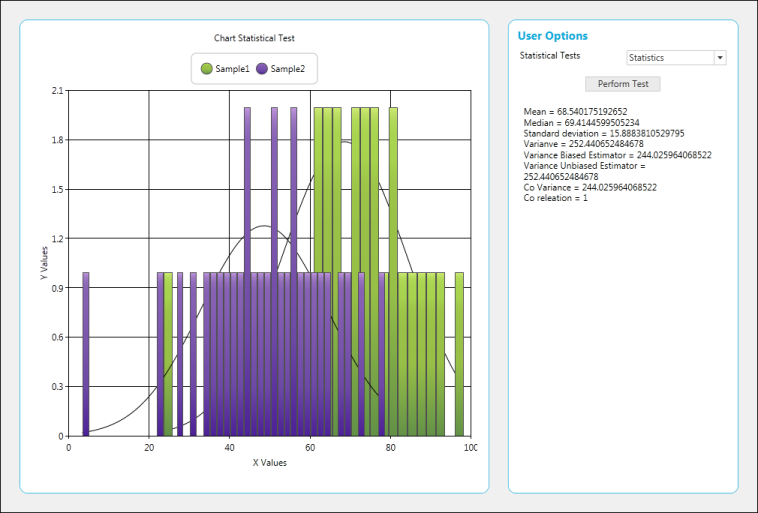## Perform ANOVA Test

• C#
• ``````AnovaResult anova = BasicStatisticalFormulas.Anova(0.05, new ChartSeries[] { series1, series2 });

result.Text = "F Ratio = " + anova.FRatio + "\n" +

"F Critical Value =" + anova.FCriticalValue + "\n" +

"Degree of Freedom Between Groups = " + anova.DegreeOfFreedomBetweenGroups + "\n" +

"Degree of Freedom within Groups = " + anova.DegreeOfFreedomWithinGroups + "\n" +

"Degree of Freedom total = " + anova.DegreeOfFreedomTotal + "\n" +

"Mean square variance beteeen groups = " + anova.MeanSquareVarianceBetweenGroups + "\n" +

"Mean square variance within groups = " + anova.MeanSquareVarianceWithinGroups + "\n" +

"Sum of square between groups = " + anova.SumOfSquaresBetweenGroups + "\n";``````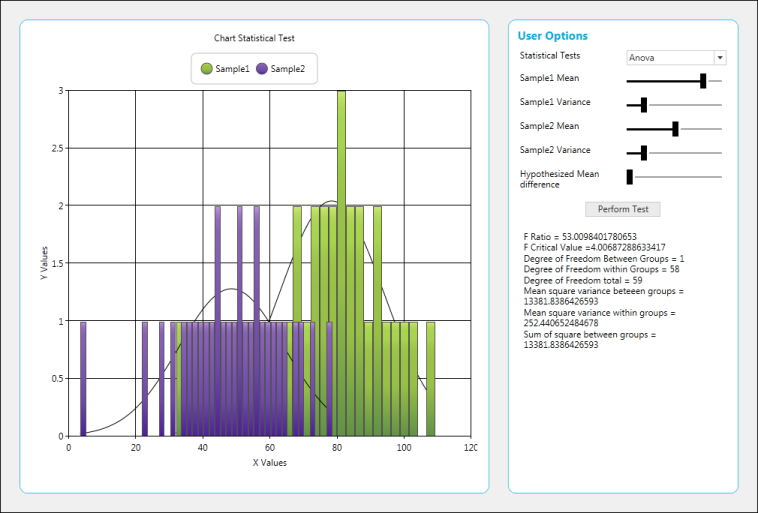## Perfrom F-Test

• C#
• ``````FTestResult ftest = BasicStatisticalFormulas.FTest(0.05, series1, series2);

result.Text = "FValue = " + ftest.FValue.ToString() + "\n" +

"F Critical Value on Tail = " + ftest.FCriticalValueOneTail.ToString() + "\n" +

"ProbabilityFOneTail = " + ftest.ProbabilityFOneTail.ToString() + "\n" +

"First Series Mean = " + ftest.FirstSeriesMean.ToString() + "\n" +

"Second Series Mean = " + ftest.SecondSeriesMean.ToString() + "\n" +

"First Series Variance = " + ftest.FirstSeriesVariance.ToString() + "\n" +

"Second Series Variance = " + ftest.SecondSeriesVariance.ToString() + "\n";``````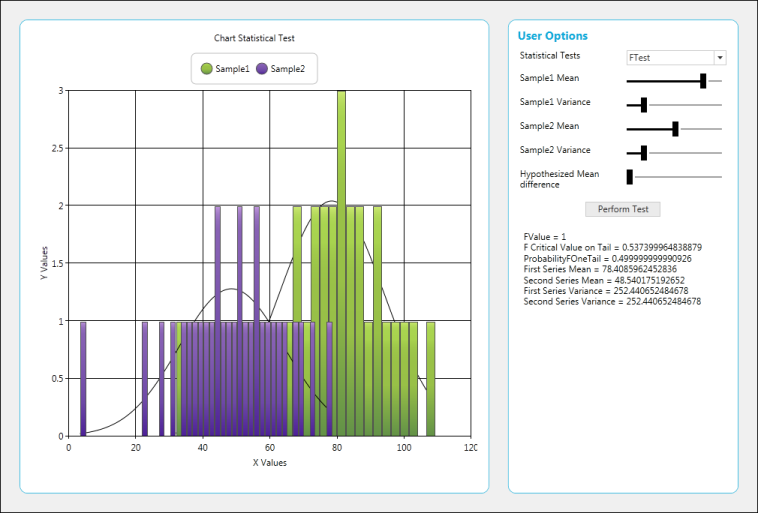## Perform T-Test

• C#
• ``````TTestResult ttest = BasicStatisticalFormulas.TTestEqualVariances(meandiff.Value, 0.1, series1, series2);

result.Text = "T Value = " + ttest.TValue.ToString() + "\n" +

"T Critical Value one Tail = " + ttest.TCriticalValueOneTail.ToString() + "\n" +

"T Critical value two Tail = " + ttest.TCriticalValueTwoTail.ToString() + "\n" +

"Probability T One Tail = " + ttest.ProbabilityTOneTail.ToString() + "\n" +

"Probability T Two Tail = " + ttest.ProbabilityTTwoTail.ToString() + "\n" +

"First Series Mean = " + ttest.FirstSeriesMean.ToString() + "\n" +

"First Series Variance = " + ttest.FirstSeriesVariance.ToString() + "\n" +

"Second Series Mean = " + ttest.SecondSeriesMean.ToString() + "\n" +

"Second Series Variance =" + ttest.SecondSeriesVariance.ToString() + "\n";``````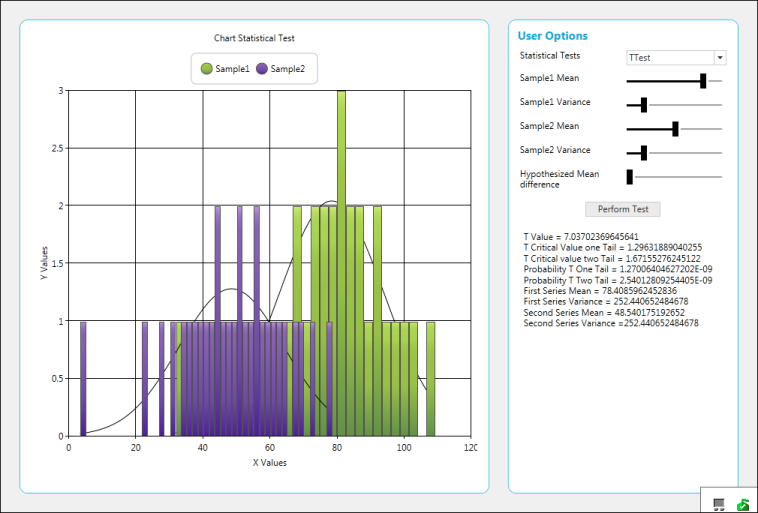## Perform Z-Test

• C#
• ``````ZTestResult ztest = BasicStatisticalFormulas.ZTest(meandiff.Value, 10, 5, 0.5, series1, series2);

result.Text = "Z Value = " + ztest.ZValue.ToString() + "\n" +

"Z Critical Value One Tail = " + ztest.ZCriticalValueOneTail.ToString() + "\n" +

"Z Critical Value Two Tail = " + ztest.ZCriticalValueTwoTail.ToString() + "\n" +

"Probability Z One Tail = " + ztest.ProbabilityZOneTail.ToString() + "\n" +

"Probability Z Two Tail = " + ztest.ProbabilityZTwoTail.ToString() + "\n" +

"First Series Mean = " + ztest.FirstSeriesMean.ToString() + "\n" +

"Second Series Mean = " + ztest.SecondSeriesMean.ToString() + "\n" +

"First Series Variance = " + ztest.FirstSeriesVariance.ToString() + "\n" +

"Second Series Variance = " + ztest.SecondSeriesMean.ToString() + "\n";``````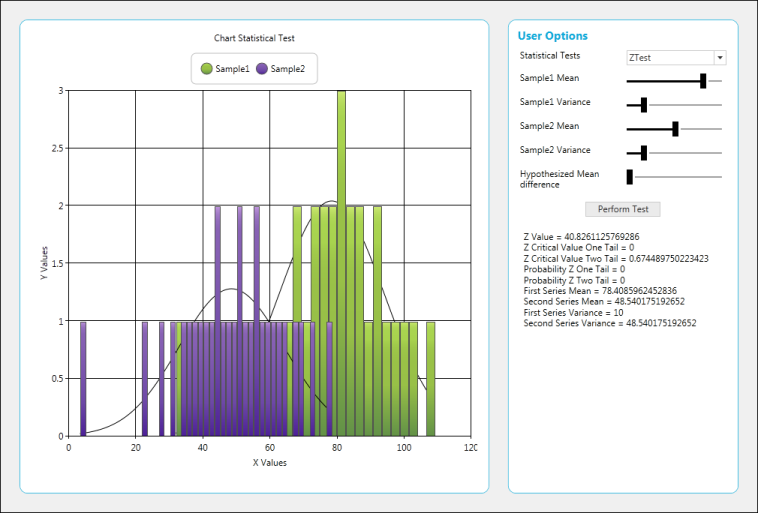## Normal Distribution

• C#
• ``value.Text = UtilityFunctions.NormalDistribution((double)probability.SelectedItem).ToString();``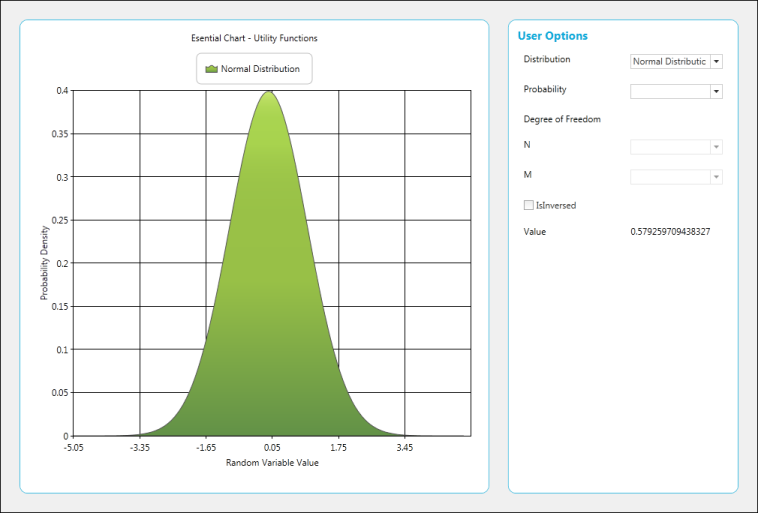## F-Cumulative Distribution

• C#
• ``value.Text = UtilityFunctions.FCumulativeDistribution((double)probability.SelectedItem, (double)n.SelectedItem, (double)m.SelectedItem).ToString();``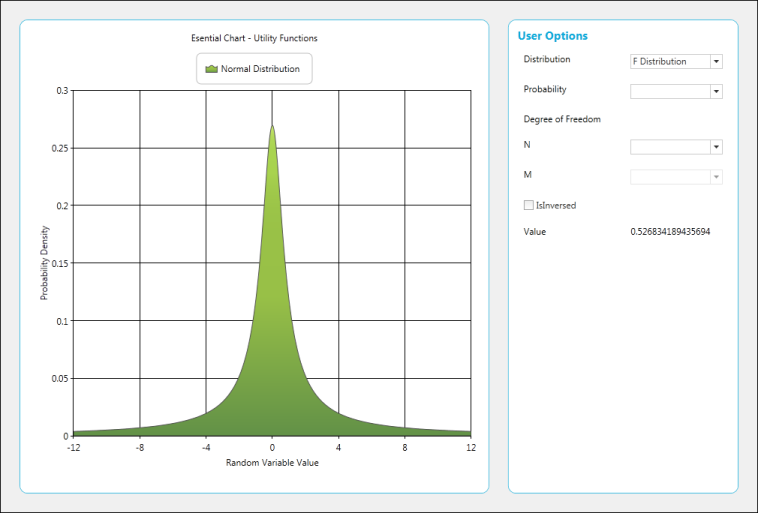## T-Cumulative Distribution

• C#
• ``value.Text = UtilityFunctions.TCumulativeDistribution((double)probability.SelectedItem, (double)n.SelectedItem, true).ToString();``